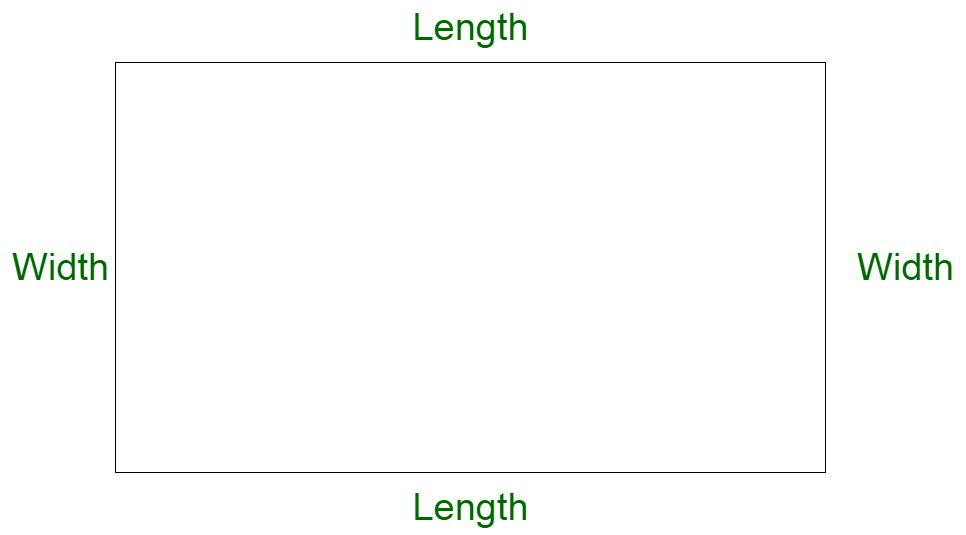# Find the length of a rectangle whose perimeter is 40 cm and base is 5 cm

• Last Updated : 20 Nov, 2021

Rectangle is a closed two-dimensional figure composed of four sides and four vertices. All angles of the rectangle are 90°. A rectangle with all sides equal is equivalent to a square. A rectangle is composed of two pairs of parallel sides, length and breadth respectively.

Properties of rectangle

• It has four sides and four angles.
• All the angles are right angles.
• Opposite sides are parallel and equal in length.

### Perimeter of Rectangle

Perimeter of the rectangle is the total summation of the boundary of all the sides of the rectangle. It is the sum of all the edge lengths of the rectangle.

Perimeter of rectangle = Sum of all sides of rectangle

Let us assume l to be the length and b to be the breadth of the rectangle.

Let us assume P to be the perimeter of the rectangle.

P = l + b + l + b

P = 2l + 2b

P = 2(l+b)### Find the length of a rectangle whose perimeter is 40 cm and base is 5 cm

Solution:

According to the problem statement, we have,

Let us assume P to be the perimeter of the rectangle and l and b to be its length and breadth respectively.

Now,

Perimeter, P = 2 (l+b)

Substituting the values,

P = 40 cm

40 = 2(l+5)

20 = l+5

l = 15 cm

Therefore, Length of the rectangle is 15 cm.

### Sample Questions

Question 1. If the perimeter of a rectangle is 100 cm and its breadth is 10 cm then find its length?

Solution:

Here we have to find the length of the rectangle when its perimeter and breadth are given,

Given:

Perimeter of rectangle = 100 cm

Breadth of the rectangle = 10 cm

We have to find

Length of the rectangle = assume ‘l’

As we know that

Perimeter of rectangle = 2×(length + breadth)

Putting the given values in the formula

100 = 2×(l + 10)

100 = 2l + 20

100 – 20 = 2l

2l = 80

l = 80/2

l = 40 cm

Therefore,

Length of the rectangle is 40 cm.

Question 2. Calculate the breadth of the rectangular park when the perimeter of the rectangle is 250 m and its length is 100 m?

Solution:

Here we have to find the breadth of the rectangle when its perimeter and length are given,

Given:

Perimeter of rectangle = 250 m

Length of the rectangle = 100 m

We have to find

Breadth of the rectangle = assume ‘b’

As we know that

Perimeter of rectangle = 2×(length + breadth)

Putting the given values in the formula

250 = 2×(100 + b)

250 = 200 + 2b

250 – 200 = 2b

2b = 50

b = 50/2

b = 25 m

Therefore,

Breadth of the rectangle is 25 m.

Question 3. If the perimeter of a rectangle is 500 cm and its length is 4 times the breadth then find its length and breadth?

Solution:

Here we have to find the length and breadth of the rectangle

We are given that the length of the rectangle is 4 times the breadth

Assume,

Length of rectangle = l

As per the question

Length of rectangle = 4 times the breadth

Length of rectangle = 4 × b

Length of rectangle = 4b

As we know that

Perimeter of rectangle = 2×(length + breadth)

Perimeter of rectangle = 2×(l + b)

Perimeter of rectangle = 2×(4b + b)

Perimeter of rectangle = 2×(5b)

500 = 10b

b = 500/10

b = 50 cm

As given in the question

Length is 4 times the breadth

Length = 4 × 50

Length = 200 cm

Therefore,

Length of rectangle is 200 cm and breadth of rectangle is 50 cm.

Question 4. Calculate the length and breadth of the rectangle if its perimeter is 1600 m and its breadth is 1/3 times its length?

Solution:

Here we have to find the length and breadth of the rectangle

We are given that the breadth of the rectangle is 1/3 times the length

Further we can say that length of a rectangle is 3 times its breadth

Assume,

Length of rectangle = l

Length of rectangle = 3 times the breadth

Length of rectangle = 3 × b

Length of rectangle = 3b

As we know that

Perimeter of rectangle = 2×(length + breadth)

Perimeter of rectangle = 2×(l + b)

Perimeter of rectangle = 2×(3b + b)

Perimeter of rectangle = 2×(4b)

1600 = 8b

b = 1600/8

b = 200 m

As given in the question

Length is 3 times the breadth

Length = 3 × 200

Length = 600 m

Therefore,

Length of rectangle is 600 m and breadth of rectangle is 200 m.

My Personal Notes arrow_drop_up